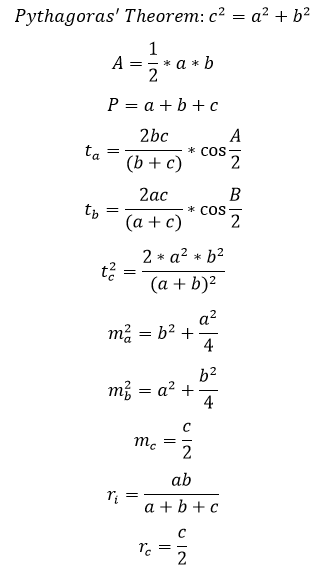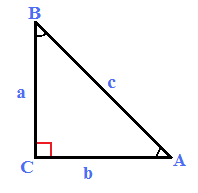# Right-angled Triangle Calculator

This CalcTown calculator calculates the various parameter related to a right-angled triangle

#### Result

m
m2
m
m
m
m
m
m
m
m
mWhere,

a = perpendicular of the right angled triangle

b = base of the right-angled triangle

c = hypotenuse of the right-angled triangle

A = area of the right-angled triangle

P = perimeter of the right-angled triangle

ta = angle bisector of side a

tb = angle bisector of side b

tc = angle bisector of side c

ma = median of side a

mb = median of side b

mc = median of side c